# Modelling of two phase flow in packed bed (continued)

• casualguitar

#### casualguitar

TL;DR Summary
Continuation of the previous post 'Modelling of two phase flow in packed bed using conservation equations'. Reason for creating new post: Not all post were visible to all responders
•berkeman

Further context:
The goal of the initial post was to model two phase flow of air through a packed bed. A number of models were developed (gradually increasing in complexity). A summary of the final model is here (I will update this summary as the model develops): https://docs.google.com/document/d/...ouid=111822275555236809516&rtpof=true&sd=true

The next stage objective will be to hopefully amend the existing mass and energy balances to include the freezing out of CO2 from the process stream

The last three posts in the original post: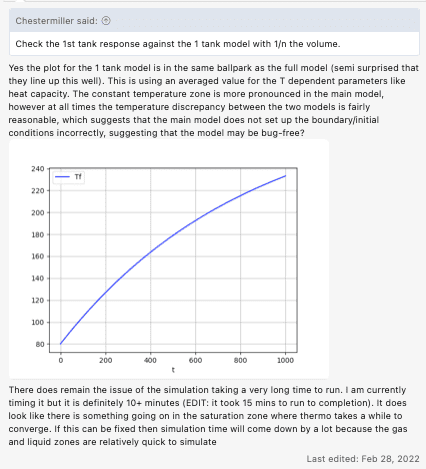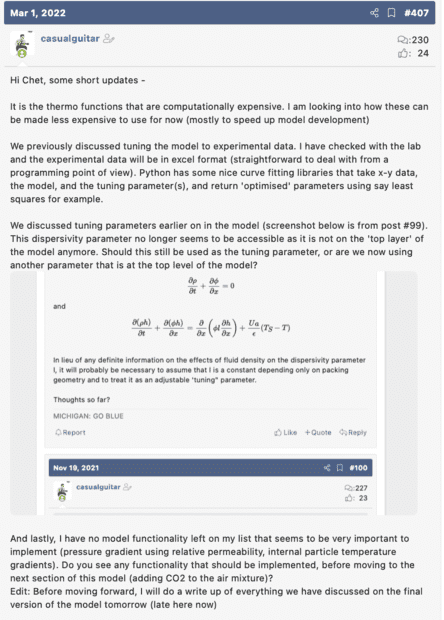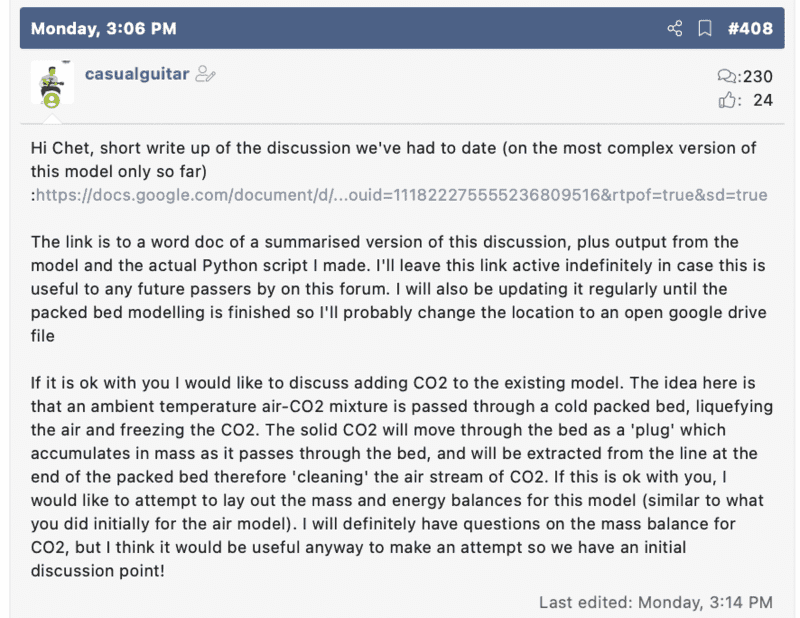Further context:
The goal of the initial post was to model two phase flow of air through a packed bed. A number of models were developed (gradually increasing in complexity). A summary of the final model is here (I will update this summary as the model develops): https://docs.google.com/document/d/...ouid=111822275555236809516&rtpof=true&sd=true

The next stage objective will be to hopefully amend the existing mass and energy balances to include the freezing out of CO2 from the process stream

The last three posts in the original post:
View attachment 298163
View attachment 298214
View attachment 298215
The tuning parameter for dispersivity is ##\Delta x=L/n##.

Regarding the CO2, what makes you think that it will move through the bed as a solid plug? Maybe it will deposit on the bed (at least temporarily). I really can't see specifically what will happen to the CO2. Is this ordinary air containing ~400 ppm CO2?

The tuning parameter for dispersivity is ##\Delta x=L/n##.

Regarding the CO2, what makes you think that it will move through the bed as a solid plug? Maybe it will deposit on the bed (at least temporarily). I really can't see specifically what will happen to the CO2. Is this ordinary air containing ~400 ppm CO2?
The CO2 would be in the range of 10-20% of the mixture by volume for example. No specified percentage yet however it would be a CO2 enriched stream i.e. much greater than 400ppm.

I don't know actually, but I think you will be right about the CO2 depositing temporarily on the bed. What I thought would happen (assuming a bed colder than the freezing point of CO2) was that the ambient CO2 enriched stream would enter the cold bed and immediately the CO2 at the 'front' of the stream would freeze. The pure air would carry on through the bed.

Then the newly entering stream - which is at ambient temperature - would vaporise the frozen CO2, and the vaporised CO2 plus the CO2 'behind' it in the stream would now be frozen/deposited slightly further downstream. This process repeats until you have a 'plug' of CO2 at the outlet of the bed.

In regards to the time though as you say, I don't know if the CO2 will be immediately vaporised, or if this freezing/boiling process will take time. If it takes longer than instantaneous times then I would guess you would see a distribution of frozen CO2 along the bed, with the most CO2 being deposited furthest down the bed, and gradually less and less CO2 being deposited towards the inlet. Sort of like the left side of a normal distribution curve (or similar).

The idea would be to freeze as much CO2 as possible in the bed in a 'plug' form. Then just sweep the bed with pure ambient temperature CO2 gas, leaving you with 'pure' air (within reason) and pure CO2 streams.

Do you agree on this much?

Papers I've read that describe the above (just two, not too many out there) -
Tuinier, M.J., van Sint Annaland, M., Kramer, G.J. and Kuipers, J.A.M., 2010. Cryogenic CO2 capture using dynamically operated packed beds. Chemical Engineering Science, 65(1), pp.114-119.

Ali, A., Maqsood, K., Syahera, N., Shariff, A.B. and Ganguly, S., 2014. Energy minimization in cryogenic packed beds during purification of natural gas with high CO2 content. Chemical Engineering & Technology, 37(10), pp.1675-1685.

This thing with the CO2 looks to me like it's going to be very tricky.

If it were me doing this, I would proceed very slowly. I would first spend a lot of time looking at the thermodynamics of the 3 component mixture (in proportions as fed to the bed), off-line from the bed model. I would first look at the vapor pressure vs temperature of O2, N2, and CO2. Then I would do phase equilibrium calculations as a function of temperature and pressure for the 2 phase (and possibly 3 phase) region. I would like to know the mole fractions of the various species in the liquid and vapor phases (and also solid phase) as a function of temperature at various fixed pressures. I would want to see how this plays out by plotting as much as I could about the mole fractions vs temperature all on single plots. But, that's just me. What were you going to do?

I would first spend a lot of time looking at the thermodynamics of the 3 component mixture (in proportions as fed to the bed), off-line from the bed model. I would first look at the vapor pressure vs temperature of O2, N2, and CO2. Then I would do phase equilibrium calculations as a function of temperature and pressure for the 2 phase (and possibly 3 phase) region. I would like to know the mole fractions of the various species in the liquid and vapor phases (and also solid phase) as a function of temperature at various fixed pressures. I would want to see how this plays out by plotting as much as I could about the mole fractions vs temperature all on single plots. But, that's just me. What were you going to do?
I can start with these yes to get an idea of the thermodynamics of the mixture. To be honest I was actually going to start with the mass and energy balance equations but that might be jumping the gun I suppose. Is it possible that the information gathered from the plots you mentioned will have an effect on the mass/energy balance equations, or are these completely independent?

I think I can get these plots done tomorrow:
- P vs Tsat for O2,N2 and CO2
- Vapor fraction vs temperature and pressure for the mixture
- Phase composition vs temperature and pressure for the mixture

Also one thing I noticed is that the triple point pressure of CO2 is about 5.2bar, meaning that we would need to operate below this pressure to allow for desublimation

I can start with these yes to get an idea of the thermodynamics of the mixture. To be honest I was actually going to start with the mass and energy balance equations but that might be jumping the gun I suppose. Is it possible that the information gathered from the plots you mentioned will have an effect on the mass/energy balance equations, or are these completely independent?

I think I can get these plots done tomorrow:
- P vs Tsat for O2,N2 and CO2
- Vapor fraction vs temperature and pressure for the mixture
- Phase composition vs temperature and pressure for the mixture

Also one thing I noticed is that the triple point pressure of CO2 is about 5.2bar, meaning that we would need to operate below this pressure to allow for desublimation
You need to get an understanding of what is going to happen before you start putting it into a more complex model.

This thing with the CO2 looks to me like it's going to be very tricky.

If it were me doing this, I would proceed very slowly. I would first spend a lot of time looking at the thermodynamics of the 3 component mixture (in proportions as fed to the bed), off-line from the bed model. I would first look at the vapor pressure vs temperature of O2, N2, and CO2. Then I would do phase equilibrium calculations as a function of temperature and pressure for the 2 phase (and possibly 3 phase) region. I would like to know the mole fractions of the various species in the liquid and vapor phases (and also solid phase) as a function of temperature at various fixed pressures. I would want to see how this plays out by plotting as much as I could about the mole fractions vs temperature all on single plots. But, that's just me. What were you going to do?

Hi Chet, temperature vs vapor pressure here for pure CO2, N2 and O2. Also included the bubble and dew point curves for a 44%CO2, 40%N2, 16% O2 mixture. These curves match up with what is expected from the CO2 property paper (ignoring the purple horizontal line for now, I haven't cleaned my code to remove that)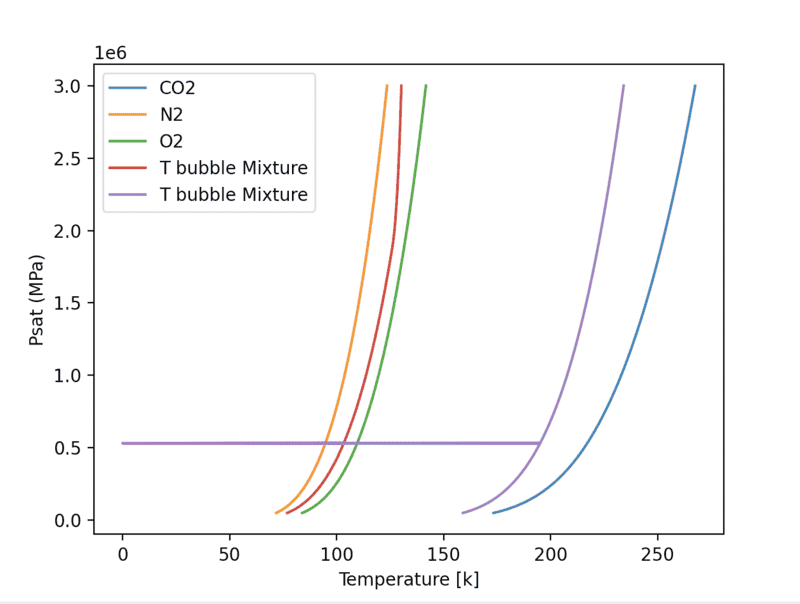There is a big gap between the liquefaction temperature of nitrogen/oxygen and that of CO2 which is probably a good thing

Just thinking some more on how best to represent the thermodynamics, it might be useful to plot the TP curves for all of N2,O2 and CO2 individually for 3 phases, just to see what phases are present for the various ranges of temperatures and pressures

I'll aim to make a TP plot for each component showing the phase present at each T,P pair, and I can overlay them actually to see where we will have more than one phase present

Hi Chet, temperature vs vapor pressure here for pure CO2, N2 and O2. Also included the bubble and dew point curves for a 44%CO2, 40%N2, 16% O2 mixture. These curves match up with what is expected from the CO2 property paper (ignoring the purple horizontal line for now, I haven't cleaned my code to remove that)
View attachment 298365
There is a big gap between the liquefaction temperature of nitrogen/oxygen and that of CO2 which is probably a good thing
Just thinking some more on how best to represent the thermodynamics, it might be useful to plot the TP curves for all of N2,O2 and CO2 individually for 3 phases, just to see what phases are present for the various ranges of temperatures and pressures

I'll aim to make a TP plot for each component showing the phase present at each T,P pair, and I can overlay them actually to see where we will have more than one phase present
The real issue is that when you have more than one phase, the liquid and solid phases will be moving through the bed slower than the vapor (i.e., lower superficial velocities). We neglected this so far in the bed model. See FLOW OF FLUIDS THROUGH POROUS MATERIALS [COLLINS, R. E.], sections on multiphase flow and relative permeabilities. This will separation effect be nasty to deal with.

Log scale plot: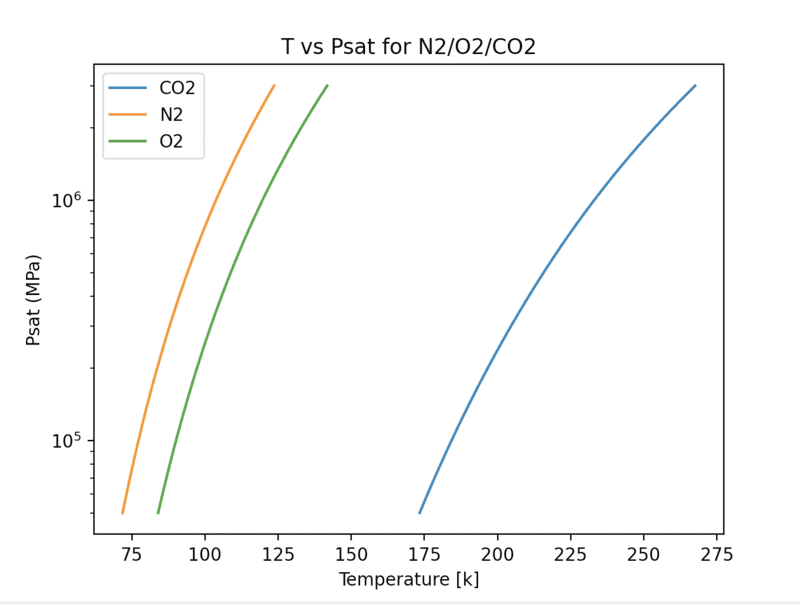Actually that horizontal purple dew point line in the previous plot happens because this is the point at which the CO2 solidifies out of the mixture (the liquid/gas correlation being used no longer applies). I'll need to switch out the liquid/gas correlation for a solid/gas one at that point

The real issue is that when you have more than one phase, the liquid and solid phases will be moving through the bed slower than the vapor (i.e., lower superficial velocities). We neglected this so far in the bed model. See FLOW OF FLUIDS THROUGH POROUS MATERIALS [COLLINS, R. E.], sections on multiphase flow and relative permeabilities. This will separation effect be nasty to deal with.
I believe I've taken a look at Collins, R.E. before regarding the use of relative permeabilities to calculate pressure drops. The math used was a level above what I'm used to so I'll need time to process this. Do you think the variation in superficial velocity across phases will have a significant impact on the model? I'll start reading this resource today

Log scale plot:
View attachment 298391
Actually that horizontal purple dew point line in the previous plot happens because this is the point at which the CO2 solidifies out of the mixture (the liquid/gas correlation being used no longer applies, hence the unusual output). I'll need to switch out the liquid/gas correlation for a solid/gas one at that point

The real issue is that when you have more than one phase, the liquid and solid phases will be moving through the bed slower than the vapor (i.e., lower superficial velocities). We neglected this so far in the bed model. See FLOW OF FLUIDS THROUGH POROUS MATERIALS [COLLINS, R. E.], sections on multiphase flow and relative permeabilities. This will separation effect be nasty to deal with.
I believe I've taken a look at Collins, R.E. before regarding the use of relative permeabilities to calculate pressure drops. The math used was a level above what I'm used to so I'll need time to process this. Do you think the variation in superficial velocity across phases will have a significant impact on the model?

Log scale plot:
View attachment 298391
The units should be Pa, not MPa.
Actually that horizontal purple dew point line in the previous plot happens because this is the point at which the CO2 solidifies out of the mixture (the liquid/gas correlation being used no longer applies, hence the unusual output). I'll need to switch out the liquid/gas correlation for a solid/gas one at that point

I believe I've taken a look at Collins, R.E. before regarding the use of relative permeabilities to calculate pressure drops. The math used was a level above what I'm used to so I'll need time to process this. Do you think the variation in superficial velocity across phases will have a significant impact on the model?
Isn't that what defines the separation you get?

Last edited:
Isn't that what defines the separation you get?
Hmm well first I will say I have started reading the flow of fluids through porous materials and it is not as far ahead of my current maths ability as I had initially thought, so I think I'll be able to discuss your above question better once I read the relevant sections

That said, I had not thought of it that way i.e. in that the separation is defined by the differences in relative permeability. In my head I had (incorrectly) assumed that:
1) The difference between the vapour (O2/N2) and liquid (O2/N2) superficial velocity, although large, would not be relevant because we are focusing on solid CO2 separation here, and
2) The superficial velocity of the solid CO2 through the bed would be so much lower than any liquid/vapour O2/N2 that the separation on this basis is not worth considering

Obviously based on my points above I do not have a good understanding of what is happening in this system in regards to why superficial velocity is important to consider here. I'll take some time to read your resource and hopefully this will be clearer

Isn't that what defines the separation you get?
Hi Chet, finished reading section 6 and 10 in the R.E. Collins reference. It was a lot more readable than I had initially thought.

Section 10 (flow with change of phase) will definitely be useful for this model, however the book only discusses liquid/vapour flow. I would guess this can be extended to include a third solid phase however I was thinking it might be better to start slowly here and first model the solidification of CO2 and the cooling of air only i.e. have the operating temperature below that of the boiling point of CO2, but above the liquefaction point of O2/N2, which would allow modelling of two phase vapour/solid flow. Does this sound reasonable?

On that front, papers that go in depth on the modelling side for this are limited. However, I did find one highly referenced paper that does do something similar, and which includes model equations:
https://www.sciencedirect.com/science/article/pii/S0009250909000852

It does not include relative permeability (or seem to directly acknowledge the difference in gas/solid superficial velocity), so I was thinking a good goal here would be to adapt this existing model to incorporate superficial velocity of the solid directly (I don't think I have the understanding yet to start developing model equations from scratch).

They model the accumulation of CO2 down the bed via a solid mass balance equation (screenshots below). This seems like a valid approach also as we could then backtrack and calculate the superficial velocity of the solid through the bed by looking at the rate of solid buildup along the bed

Do you think it is reasonable to begin by using this existing model as a reference? If so I can synthesise/summarise their model and equations today/tomorrow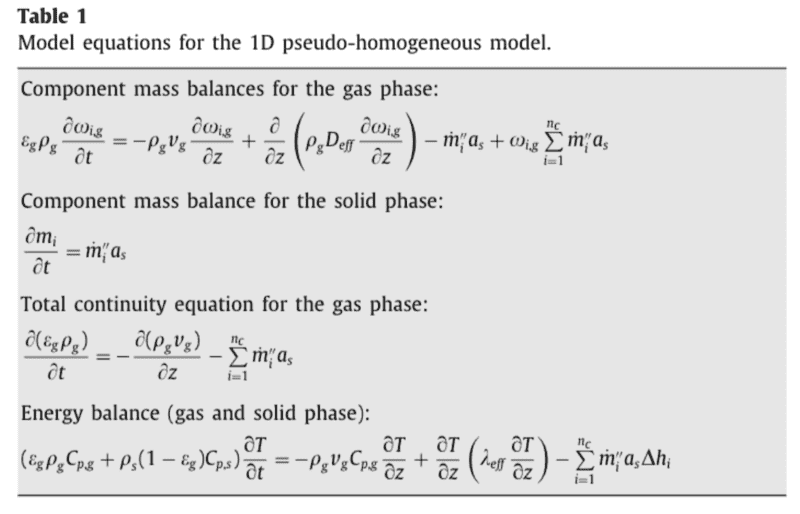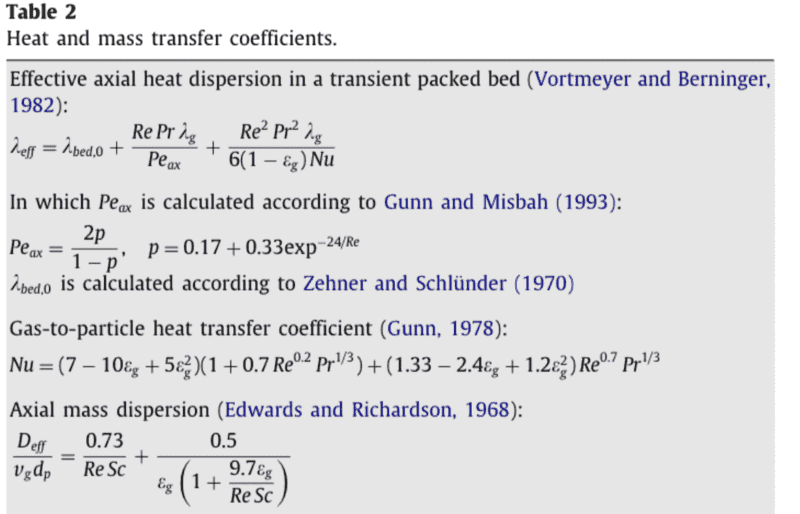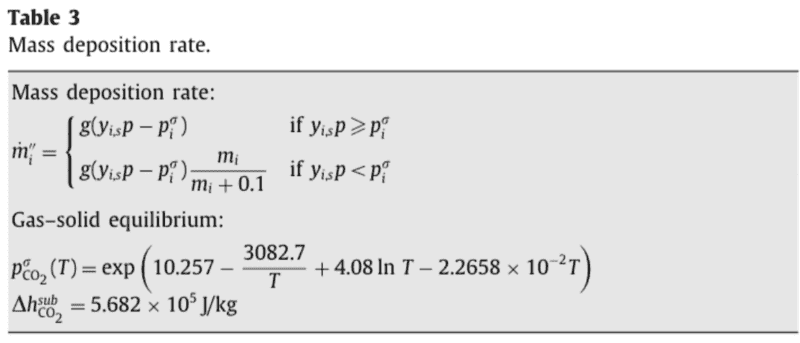Note: I have not included the nomenclature here as I'm just including these screenshots to give an idea of what their model looks like

Log scale plot:
View attachment 298391
Let's see the solid-gas region for CO2 also included on this plot.

Hi Chet, finished reading section 6 and 10 in the R.E. Collins reference. It was a lot more readable than I had initially thought.

Section 10 (flow with change of phase) will definitely be useful for this model, however the book only discusses liquid/vapour flow. I would guess this can be extended to include a third solid phase however I was thinking it might be better to start slowly here and first model the solidification of CO2 and the cooling of air only i.e. have the operating temperature below that of the boiling point of CO2, but above the liquefaction point of O2/N2, which would allow modelling of two phase vapour/solid flow. Does this sound reasonable?
Yes. But, if this were an ideal gas mixture (which it is not), the partial pressure of the CO2 in the entering stream would have to be lower than(or equal to) the CO2 triple point temperature. Then the CO2 would condense out as a solid first. Assuming that the air is not soluble in the solid CO2, the cooling could continue at lower temperatures until liquid started forming. It is not clear thermodynamically what would happen next (without experimental information on the 3 component behavior). Also, there is a question of what is present in the bed to begin with.
On that front, papers that go in depth on the modelling side for this are limited. However, I did find one highly referenced paper that does do something similar, and which includes model equations:
https://www.sciencedirect.com/science/article/pii/S0009250909000852
I don't have free access to this paper, because I am not in any organization that subscribes to the journal.
It does not include relative permeability (or seem to directly acknowledge the difference in gas/solid superficial velocity), so I was thinking a good goal here would be to adapt this existing model to incorporate superficial velocity of the solid directly (I don't think I have the understanding yet to start developing model equations from scratch).
It must be assuming that the solid is totally immobilized on the bed.
They model the accumulation of CO2 down the bed via a solid mass balance equation (screenshots below). This seems like a valid approach also as we could then backtrack and calculate the superficial velocity of the solid through the bed by looking at the rate of solid buildup along the bed
It must be assuming that the solid is totally immobilized on the bed.
Do you think it is reasonable to begin by using this existing model as a reference? If so I can synthesise/summarise their model and equations today/tomorrow

View attachment 298442
View attachment 298443
View attachment 298444
Note: I have not included the nomenclature here as I'm just including these screenshots to give an idea of what their model looks like
This model is definitely assuming that the solid component is (axially) immobilized on the bed. Because of my inaccessibility to the paper, I will need to know the nomenclature. I would also like to see their definition of Re and Nu. Thanks.

Hi Chet, I was away at a neighbours wedding (which was up the west coast of Ireland). I'm back on this now
Let's see the solid-gas region for CO2 also included on this plot.
Can do. Actually I did not see this functionality in the thermo library so I checked with the owner and they confirmed that it is not something that thermo currently does. I am sure the CO2 sublimation curve exists in literature so I could create this manually as a polynomial for example? The issue with this is that it will only be correct for pure CO2 and possibly not for various CO2/air mixtures i.e. I now cannot see how the CO2 sublimation curve moves in the presence of air for example. Adding this functionality in would be a large job I would imagine so maybe for now its best to just take the sublimation curve as a polynomial from literature/literature data, assuming pure CO2? Is it an unreasonably large piece of work to remove the use of the thermo library completely and to use our own correlations for these curves (and therefore for the calculation of enthalpy, temperature from PH, etc, all of those small calculations that thermo currently takes care of). Or can we just use our own sublimation curve here and maintain thermo for everything else?

It must be assuming that the solid is totally immobilized on the bed.
If we are assuming that the solid is totally immobilised, then why would it be important to know the superficial velocity of the air? So that we can find the rate that the 'immobilised' solid moves through the bed?

Because of my inaccessibility to the paper, I will need to know the nomenclature. I would also like to see their definition of Re and Nu. Thanks.
Sure, screenshot here of the nomenclature (PDF of the paper attached to this comment for reference):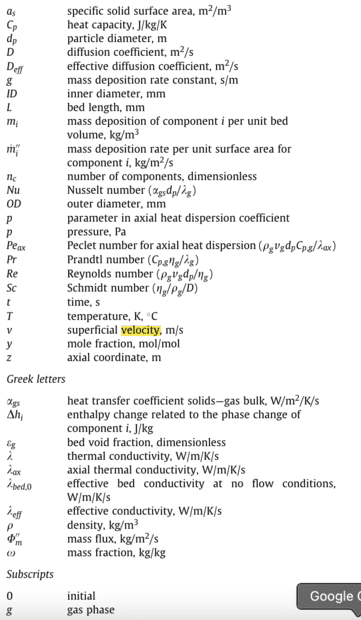Definitions of Re and Nu are from the Dixon and Cresswell reference we previously used: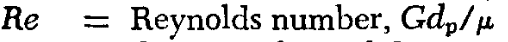And here is the Nu we previously used:
https://www.physicsforums.com/attachments/298685
If the above is reasonable I will start tomorrow first thing on finding a solid/vapour curve (or curve data to make a curve), followed by continuing on my understanding of that paper I linked

#### Attachments

Actually no particular need to get the sublimation curve from literature. This paper already supplies it in table 3: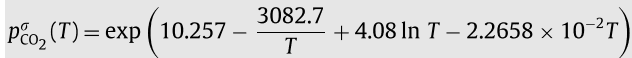I'll see what this looks like with the liquid vapour curve

Actually no particular need to get the sublimation curve from literature. This paper already supplies it in table 3:
View attachment 298713

I'll see what this looks like with the liquid vapour curve
I've added the vapour/liquid and vapour/solid curves to the same plot: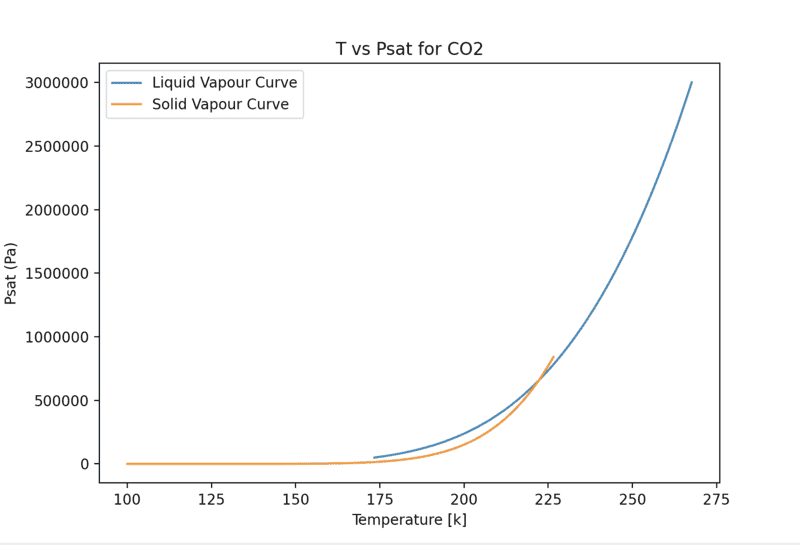As you can see I have plotted both curves slightly outside their respective 'valid' ranges to illustrate something.

The sublimation curve is really only valid up to about 222K (where it overlaps the VL curve), and the VL curve is only valid down to this temperature (the triple point temperature). However, if you extend the VL plot down through the 'solid' CO2 range, it maps relatively well to the solid/vapour curve. So rather than adding an external polynomial here to check CO2 phase, we could possibly use the extended VL curve to check if the phase is solid or vapour

I will start 'flowcharting' the model from the attached paper above now (mostly to get an idea of how this solid deposition stuff is going to be modeled)

I've added the vapour/liquid and vapour/solid curves to the same plot:
View attachment 298720
As you can see I have plotted both curves slightly outside their respective 'valid' ranges to illustrate something.

The sublimation curve is really only valid up to about 222K (where it overlaps the VL curve), and the VL curve is only valid down to this temperature (the triple point temperature). However, if you extend the VL plot down through the 'solid' CO2 range, it maps relatively well to the solid/vapour curve. So rather than adding an external polynomial here to check CO2 phase, we could possibly use the extended VL curve to check if the phase is solid or vapour

I will start 'flowcharting' the model from the attached paper above now (mostly to get an idea of how this solid deposition stuff is going to be modeled)
Please replot this with a logarithmic scale for pressure. You will see that there are substantial differences between the vapor-liquid curve and the vapor-solid curve at temperature significantly below the triple point.

I've looked over the paper you sent, and get the gist of what they are doing. In terms of the energy balance, they assume that the bed and gas are everywhere at the same temperature (equivalent to an infinite heat transfer coefficient between the bed and gas), but then consider a kind of Taylor dispersion axially, based on heat transfer coefficient between the bed and the gas. I guess this might be an OK approximation, but I don't like it.

There seems to me to be a big difference between what they are modeling and what you are. They are assuming that the pressure in the bed is close to atmospheric, so that ideal gas behavior can be assumed for the gas phase. You are assuming that the pressure in the bed is much higher (30 bars) where the behavior will decidedly be non-ideal. This will complicate the analysis of the de-sublimination behavior, since you will have to consider CO2 fugacity (including in the mass transfer behavior). Probably, even at 1 bar, they should also be doing this at the low temperatures involved. Thoughts on this?

I've looked over the paper you sent, and get the gist of what they are doing. In terms of the energy balance, they assume that the bed and gas are everywhere at the same temperature (equivalent to an infinite heat transfer coefficient between the bed and gas), but then consider a kind of Taylor dispersion axially, based on heat transfer coefficient between the bed and the gas. I guess this might be an OK approximation, but I don't like it.
Understood I think. So the purpose of this infinite heat transfer coefficient assumption would be to remove the need for separate gas and bed energy balances i.e. simplifying by removing one energy balance PDE? In our previous model the bed and fluid temperatures tend to be fairly close, so I'm not sure how much going with the infinite HTC assumption would affect the model

If I understand the axial Taylor dispersion correctly, this means that they are assuming that the total solid CO2 buildup is just equal to the mass deposition rate times the total surface area in an element. What would be the alternative here? Could the use of a 'mass deposition rate' term be avoided, and instead a vapour/solid equilibrium calculation be done at each position along the bed?

There seems to me to be a big difference between what they are modeling and what you are. They are assuming that the pressure in the bed is close to atmospheric, so that ideal gas behavior can be assumed for the gas phase. You are assuming that the pressure in the bed is much higher (30 bars) where the behavior will decidedly be non-ideal. This will complicate the analysis of the de-sublimination behavior, since you will have to consider CO2 fugacity (including in the mass transfer behavior). Probably, even at 1 bar, they should also be doing this at the low temperatures involved. Thoughts on this?
To clarify yes in the previous model the operating pressure was 30 bar, however here for the separation of CO2 from air there is no required pressure in place yet, so we could choose to go with a pressure below the triple point pressure (below approx 5.2bar), that would allow for desublimation.

If we are not considering the phase change of air here (vapour phase only), we could also return back to the temperature derivative approach (as is used here) which may simplify the model slightly. Also, given the pressure will be a low lower here, we may be able to reasonably assume that some parameters like heat capacity will be constant (which would simplify the model again and speed it up if it meant we could avoid using the thermo library for the most part)

Side note - actually as far as I know the liquefaction of air is much more efficient at higher pressures. I am not sure if this applies to packed bed systems. If it does, then it would mean that operating below the triple point pressure of CO2 (to allow for desublimation) would mean lower efficiency liquefaction of air

Log plot here: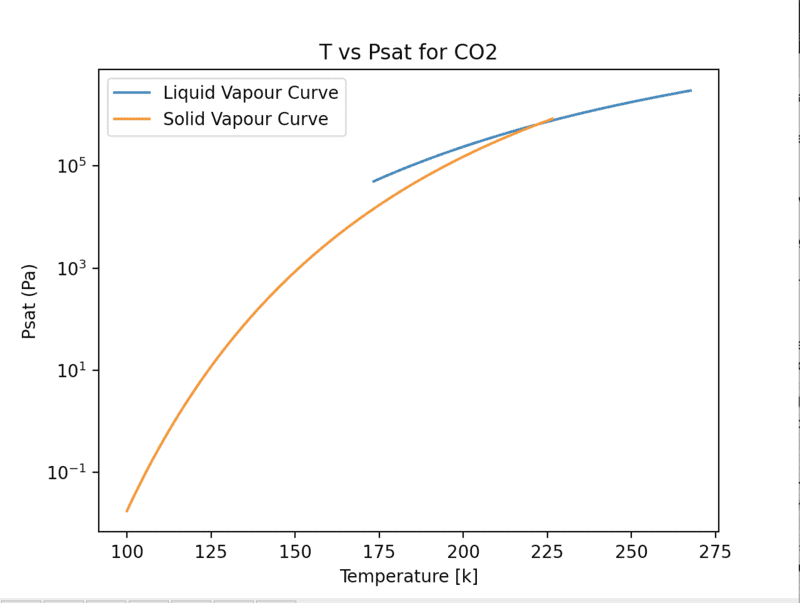Understood I think. So the purpose of this infinite heat transfer coefficient assumption would be to remove the need for separate gas and bed energy balances i.e. simplifying by removing one energy balance PDE? In our previous model the bed and fluid temperatures tend to be fairly close, so I'm not sure how much going with the infinite HTC assumption would affect the model
Is this CO2 model of yours supposed to be a totally separate model, or do you envision using that same model structure for the CO2 as for the air liquification model? Or are you going to carry along two separate model structures and compare predictions for both of them for both CO2 and air? What exactly are you trying to do?
If I understand the axial Taylor dispersion correctly, this means that they are assuming that the total solid CO2 buildup is just equal to the mass deposition rate times the total surface area in an element. What would be the alternative here? Could the use of a 'mass deposition rate' term be avoided, and instead a vapour/solid equilibrium calculation be done at each position along the bed?
This Taylor dispersion has nothing to do with CO2 deposition. It is related to the dispersion of heat along the bed.

In this model and physical situation, CO2 is being deposited on the cold solid bed (where it is immobile), and, at subsequent times, when the hotter gas feed sublimates the deposited CO2, the CO2 becomes more concentrated in the trailing gas than in the entering feed. In this way, we get a CO2 separation process.

It seems that for the water, the same immobilization is being assumed in their model to concentrate the water. They seem to assume that the two depositions are independent, even though they have some common region of deposition.

In our model, what we are doing is assuming that the liquified air travels along with the overall fluid stream, rather than any of it depositing and immobilizing on the bed. Which do you think is a more accurate rendition of reality?
To clarify yes in the previous model the operating pressure was 30 bar, however here for the separation of CO2 from air there is no required pressure in place yet, so we could choose to go with a pressure below the triple point pressure (below approx 5.2bar), that would allow for desublimation.
They are operating at atmospheric pressure, and, in their calculations, the partial pressure of CO2 in the feed is only 0.2 bars.

Is this CO2 model of yours supposed to be a totally separate model, or do you envision using that same model structure for the CO2 as for the air liquification model? Or are you going to carry along two separate model structures and compare predictions for both of them for both CO2 and air?
It is a separate model. However if it so happens that using the same structure as the previous model is useful for this model also then great. But no my initial thought is that it would be better to not force the models to use the same structure if there is a better or more natural alternative

What exactly are you trying to do?
I haven't fully defined this yet, however the core idea may be to answer the question:
How good can cryogenic packed beds get at separating CO2 from air (and how do they compare to alternatives)?'.

Is this a reasonable question to answer?

In this model and physical situation, CO2 is being deposited on the cold solid bed (where it is immobile), and, at subsequent times, when the hotter gas feed sublimates the deposited CO2, the CO2 becomes more concentrated in the trailing gas than in the entering feed. In this way, we get a CO2 separation process.

It seems that for the water, the same immobilization is being assumed in their model to concentrate the water. They seem to assume that the two depositions are independent, even though they have some common region of deposition.
Understood

In our model, what we are doing is assuming that the liquified air travels along with the overall fluid stream, rather than any of it depositing and immobilizing on the bed. Which do you think is a more accurate rendition of reality?
Hmm I think our previous model is less accurate here because we don't consider that superficial velocity through the bed is dependent on phase. I'm not sure how much their assumption that the two depositions are independent would affect the model however I would imagine it is significant. So it seems to be correct to say the 'best' answer is somewhere between the two approaches:
1) Accounting for the differences in superficial velocities depending on phase
2) Dealing with the two dependent depositions which would result in non-perfect deposition at any point along the bed i.e. the deposition would be limited by bed surface area

It seems we will definitely need to deal with the phase superficial velocity differences in this model. Luckily we won't have to deal with two dependent depositions as we do not have water in the feed (or any other solidifying component).

So to deal with the superficial velocity my thoughts are that we could:
1) go back and add this functionality to the previous model
2) make a very basic model that just tracks superficial velocity along the bed as a function of temperature for a single component fluid
3) 'ignore' this for now and start developing a simple version of the CO2 model, considering superficial velocity/relative permeability once the model is slightly more developed

I think either 2 or 3 here are the most suitable approaches. I'm happy with either. I would think #3 is probably best.

Either way, both approaches will require that I understand the Tuinier et al paper so I will aim to get a summary/calculation flow of this model this evening.

Either way, both approaches will require that I understand the Tuinier et al paper so I will aim to get a summary/calculation flow of this model this evening.
Hi Chet. I read through the paper this evening. I have some sections of the paper that I do not understand and would like to clear up if possible

"The rate of mass deposition and sublimation of CO2 was assumed to be proportional to the local deviation from the phase equilibrium"
Is it correct to say that they are saying that the rate of mass deposition/sublimation is proportional to the 'distance' between the partial pressure of the CO2 and the saturation pressure i.e. the further the partial pressure from the saturation pressure, the faster it changes phase?

"The velocity of the condensing front is inherently faster than the velocity of the evaporating front, due to the opposed enthalpies involved in the condensation/evaporation."
It makes sense to me that the vapour phase (condensing) would be faster than the liquid phase (liquid) due to the density difference, however I'm not sure what the need for mentioning 'opposed enthalpies' is here. Why is opposed enthalpies important here?

The model equations: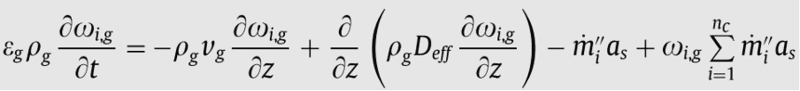The rate of increase of mass fraction of a component i is equal to the the rate of change of mass fraction of the component along the bed due to advection plus the rate of mass diffusion along the bed minus the mass lost to the solid phase, plus the gas gained from the solid phase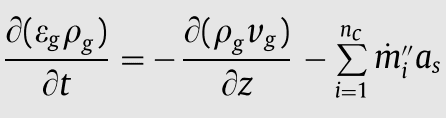I think this is the same as above just on a 'total' basis rather than component basis?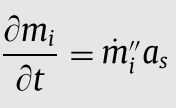This is just the rate of increase of solid component is equal to the mass deposition rate times the specific surface area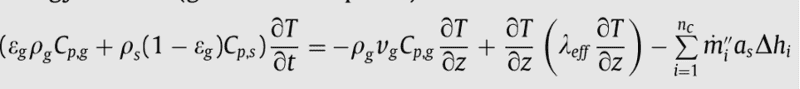This is a gas and solid total energy balance in the one equation . Anyway I think it means - the rate of increase of energy is equal to the loss due to convection plus the rate of conduction along the bed minus the heat lost/gained by change in phase

Two notes here:

1) I used the term advection for the mass balance and convection for the energy balance. Is this correct?

2) Actually, looking at the energy balance and the way they have 'tacked on' the phase change term at the end of the energy balance, this is exactly the question I was trying to ask about in my initial post on stack exchange (before we moved to this forum). The papers I was reading (and did not understand) all dealt with single phase only, and I was wondering if you could reasonably add on the 'phase change term' like this in the energy balance. I suppose this answers the original question. Looking back now I was not nearly in a position to understand that at at the time though (and arguably still am not). Again hugely appreciated for carrying me through this! I will get to the point of being able to do this independently. I'm not there yet but that's the idea and I'm motivated to get there

It is a separate model. However if it so happens that using the same structure as the previous model is useful for this model also then great. But no my initial thought is that it would be better to not force the models to use the same structure if there is a better or more natural alternative

I haven't fully defined this yet, however the core idea may be to answer the question:
How good can cryogenic packed beds get at separating CO2 from air (and how do they compare to alternatives)?'.
Are you sure you are not primarily interested in separating CO2 from combustion products (not air).
Hmm I think our previous model is less accurate here because we don't consider that superficial velocity through the bed is dependent on phase.
We are assuming that the condensed liquid travels at the same superficial velocity as the gas and, at least tentatively, that it is not deposited on- or slowed down by the bed. Your calculations have shown that this is not too bad an assumption since all the conversion from vapor to liquid occurs over a very short section (only a few tanks) of the bed.
I'm not sure how much their assumption that the two depositions are independent would affect the model however I would imagine it is significant. So it seems to be correct to say the 'best' answer is somewhere between the two approaches:
1) Accounting for the differences in superficial velocities depending on phase
2) Dealing with the two dependent depositions which would result in non-perfect deposition at any point along the bed i.e. the deposition would be limited by bed surface area

It seems we will definitely need to deal with the phase superficial velocity differences in this model. Luckily we won't have to deal with two dependent depositions as we do not have water in the feed (or any other solidifying component).

So to deal with the superficial velocity my thoughts are that we could:
1) go back and add this functionality to the previous model
2) make a very basic model that just tracks superficial velocity along the bed as a function of temperature for a single component fluid
3) 'ignore' this for now and start developing a simple version of the CO2 model, considering superficial velocity/relative permeability once the model is slightly more developed
I assume you are never going to have both air- and CO2 condensation/desublimation in the same model. So the existing model would handle air condensation along and a new model would focus on CO2 separation from a combustion gas. Is this a possibility in your thinking?

In the latter case, water condensation would also have to be handled in some way, in combination with the CO2 deposition.

So there would be two models:
1. Air condensation model without CO2 and water
2. Combustion gas separation model without N2 and O2 condensation, but with water condensation and CO2 desublimation.

Model 1 would basically be the existing model, featuring
1. High pressure, non-ideal thermodynamics for the gas phase
2. No deposition of solid or liquid onto or off of the solid bed (No fluid to solid interphase mass transfer)
3. Separate temperatures for the solid and fluid phases

Model 2, the CO2 separation model, would include
1. Low pressure, ideal gas thermodynamics for the gas phase
2. Deposition of CO2 (and possibly water) from the gas to the solid and CO2 sublimation from the solid to the gas
3. Relatively low CO2 concentrations, so it can be treated as a trace species, without counterdiffusion mass transfer effects.
4. Separate temperatures for the solid and fluid phases (even though the Tuinier model lumps the phases to a single temperature). I think this flexibility should be retained.

Thoughts?

I have lots to say about the Tuinier model, but am running short in time now, so I will address this later.

Are you sure you are not primarily interested in separating CO2 from combustion products (not air).
I was initially thinking just CO2 but actually the applicability of that is quite low. Combustion products does seem a lot more interesting so yes let's go with CO2 and water

I assume you are never going to have both air- and CO2 condensation/desublimation in the same model. So the existing model would handle air condensation along and a new model would focus on CO2 separation from a combustion gas. Is this a possibility in your thinking?
Yes it seems so given that the CO2 model is only possible at low pressure and the air liquefaction model is at high pressure. So yes this does sound nice - one model for air condensation and one model for CO2 separation from a combustion gas. Sounds good

Model 1 would basically be the existing model, featuring
1. High pressure, non-ideal thermodynamics for the gas phase
2. No deposition of solid or liquid onto or off of the solid bed (No fluid to solid interphase mass transfer)
3. Separate temperatures for the solid and fluid phases

Model 2, the CO2 separation model, would include
1. Low pressure, ideal gas thermodynamics for the gas phase
2. Deposition of CO2 (and possibly water) from the gas to the solid and CO2 sublimation from the solid to the gas
3. Relatively low CO2 concentrations, so it can be treated as a trace species, without counterdiffusion mass transfer effects.
4. Separate temperatures for the solid and fluid phases (even though the Tuinier model lumps the phases to a single temperature). I think this flexibility should be retained.

Thoughts?
Model 1 I fully agree with and I think we have done this for the most part

Model 2:
- The ideal gas phase thermodynamic assumption could be interesting in that it would allow for some hand calculations to be done (I have avoided this almost completely by using the thermo library up until now). Also using hand calculations could result in an improvement on the model run time compared to the liquefaction model in which the thermo library is used
- If this model was to be of interest in a 'practical' setting i.e. actually using a cryogenic temperature packed bed to clean a combustion gas, then it seems that it would need to work for higher concentrations of CO2 also. We could split model 2 into: a) CO2 as a trace species (not considering counterdiffusion mass transfer) and b) CO2 as a non-trace species (considering counterdiffusion mass transfer)?

So if we are going with combustion gas CO2 separation rather than just CO2, we can use the Tuinier model for the most part with some changes (like not lumping the phases into one temperature). If this is the case, then I could replicate the Tuinier model in code as a comparison with our model (with the changes)

Side note:
I don't know if I have actually mentioned the broader area I am working in for my PhD but for context it is energy storage systems and currently liquid air energy storage systems. The packed bed is possibly a better way to liquefy air than the current liquefaction cycles (Linde, Claude, etc), and the possibility of the packed bed being multifunctional (liquefaction, combustion gas cleaning) is also interesting

Maybe the next step here is to adjust the existing model equations to account for the separate solid and fluid temperatures? If so I can attempt this

Last edited:
I was initially thinking just CO2 but actually the applicability of that is quite low. Combustion products does seem a lot more interesting so yes let's go with CO2 and water

Yes it seems so given that the CO2 model is only possible at low pressure and the air liquefaction model is at high pressure. So yes this does sound nice - one model for air condensation and one model for CO2 separation from a combustion gas. Sounds good

Model 1 I fully agree with and I think we have done this for the most part

Model 2:
- The ideal gas phase thermodynamic assumption could be interesting in that it would allow for some hand calculations to be done (I have avoided this almost completely by using the thermo library up until now). Also using hand calculations could result in an improvement on the model run time compared to the liquefaction model in which the thermo library is used
- If this model was to be of interest in a 'practical' setting i.e. actually using a cryogenic temperature packed bed to clean a combustion gas, then it seems that it would need to work for higher concentrations of CO2 also. We could split model 2 into: a) CO2 as a trace species (not considering counterdiffusion mass transfer) and b) CO2 as a non-trace species (considering counterdiffusion mass transfer)?
What is the typical CO2 mole fraction in combustion gases? My guess is that it is pretty low because of the nitrogen. The Tuinier model calculations were at an inlet CO2 mole fraction of 0.2.
Hi Chet. I read through the paper this evening. I have some sections of the paper that I do not understand and would like to clear up if possible

"The rate of mass deposition and sublimation of CO2 was assumed to be proportional to the local deviation from the phase equilibrium"
Is it correct to say that they are saying that the rate of mass deposition/sublimation is proportional to the 'distance' between the partial pressure of the CO2 and the saturation pressure i.e. the further the partial pressure from the saturation pressure, the faster it changes phase?
The driving force for mass transfer is the difference between the partial pressure of CO2 in the bulk and the saturation vapor pressure of CO2 at the interface. This is a standard way of handling the mass transfer at a phase boundary.
"The velocity of the condensing front is inherently faster than the velocity of the evaporating front, due to the opposed enthalpies involved in the condensation/evaporation."
It makes sense to me that the vapour phase (condensing) would be faster than the liquid phase (liquid) due to the density difference, however I'm not sure what the need for mentioning 'opposed enthalpies' is here. Why is opposed enthalpies important here?
I have no idea what this is about. It looks like they use a fudge factor to make the rate of desublimation higher than the rate of sublimation: a factor of 1.0 vs a factor of ##\frac{m}{m+0.1}##, where the 0.1 represents ##0.1 kg/m^3##.

Mass balance equations:
For each individual component:
View attachment 298829
Overall mass balance:
View attachment 298830
If we take this overall mass balance, multiply it by the species mass fraction in the gas ##\omega_{i,g}##, and add the resulting equation to the species mass balance, we obtain a new version of the species mass balance equation:
$$\frac{\partial (\epsilon_g\rho_g\omega_{i,g})}{\partial t}+\frac{\partial (v_g\rho_g\omega_{i,g})}{\partial z}=\frac{\partial}{\partial z}\left(\rho_gD_{eff}\frac{\partial \omega_{i,g}}{\partial z}\right)-\dot{m}_i^"a_s$$This would usually be referred to as the "divergence form" of the species mass balance equation, and is beginning to look in form like the heat balance equation in our other model. There is axial dispersion of the species as well as deposition of the species onto the solid bed.

For the solid bed, the mass balance equation would read:
View attachment 298831

Is this OK for you so far. I will address the heat balance equations for the gas and the bed in my next posting, treating the two phases separately, including heat transfer between the gas and the bed and relative distribution of the heat of desublimation between the two. The result will be much closer to the model we already have in hand.

Last edited:
By the way, for this CO2 model, there is an overwhelming advantage (that Tuinier missed out on) for working in terms of molar flow rates and mole fractions, rather than mass flow rates and mass fractions. I will illustrate that in the subsequent development I present.

What is the typical CO2 mole fraction in combustion gases? My guess is that it is pretty low because of the nitrogen. The Tuinier model calculations were at an inlet CO2 mole fraction of 0.2.

The driving force for mass transfer is the difference between the partial pressure of CO2 in the bulk and the saturation vapor pressure of CO2 at the interface. This is a standard way of handling the mass transfer at a phase boundary.

I have no idea what this is about. It looks like they use a fudge factor to make the rate of desublimation higher than the rate of sublimation: a factor of 1.0 vs a factor of ##\frac{m}{m+0.1}##, where the 0.1 represents ##0.1 kg/m^3##.

Mass balance equations:
For each individual component:
View attachment 298829
Overall mass balance:
View attachment 298830
If we take this overall mass balance, multiply it by the species mass fraction in the gas ##\omega_{i,g}##, and add the resulting equation to the species mass balance, we obtain a new version of the species mass balance equation:
$$\frac{\partial (\epsilon_g\rho_g\omega_{i,g})}{\partial t}+\frac{\partial (v_g\rho_g\omega_{i,g})}{\partial z}=\frac{\partial}{\partial z}\left(\rho_gD_{eff}\frac{\partial \omega_{i,g}}{\partial z}\right)-\dot{m}_i^"$$This would usually be referred to as the "divergence form" of the species mass balance equation, and is beginning to look in form like the heat balance equation in our other model. There is axial dispersion of the species as well as deposition of the species onto the solid bed.

For the solid bed, the mass balance equation would read:
View attachment 298831

Is this OK for you so far. I will address the heat balance equations for the gas and the bed in my next posting, treating the two phases separately, including heat transfer between the gas and the bed and relative distribution of the heat of desublimation between the two. The result will be much closer to the model we already have in hand.
Mostly good so far.

It took me a while to spot but yes I see now you've done a 'reverse chain rule' to get to the divergence form of the species mass balance after you add the component and total mass balances. I think there is an ##a_{s}## term missing at the end of the equation

Two short questions:
1) Why do you add the species and total mass balances? This seems unusual to me in that it doesn't seem like it would have physical significance. What does adding the species and total mass balances give you, from a physical meaning perspective? You mention it actually gives you the species mass balance back in divergence form, but I don't yet see how species + total = species, if you understand my question?
2) Why do you multiply across by ##\omega_{i,g}##? Are you seeing in advance that this will lead you to be able to do a 'reverse chain rule' and get to the divergence form of the species mass balance? If so, I guess knowing to do something like this comes from experience?

Besides this I'm good to move on to the heat balance

Mostly good so far.

It took me a while to spot but yes I see now you've done a 'reverse chain rule' to get to the divergence form of the species mass balance after you add the component and total mass balances. I think there is an ##a_{s}## term missing at the end of the equation

Two short questions:
1) Why do you add the species and total mass balances? This seems unusual to me in that it doesn't seem like it would have physical significance. What does adding the species and total mass balances give you, from a physical meaning perspective? You mention it actually gives you the species mass balance back in divergence form, but I don't yet see how species + total = species, if you understand my question?
You have it backwards. The original form of the species mass balance equation they derived (using a control volume approach) was the equation I wrote down (not shown in their development). They obtained their present form of the species mass balance equation by multiplying the total mass balance by the mass fraction of the species and subtracting it from the species mass balance I wrote down.
2) Why do you multiply across by ##\omega_{i,g}##? Are you seeing in advance that this will lead you to be able to do a 'reverse chain rule' and get to the divergence form of the species mass balance? If so, I guess knowing to do something like this comes from experience?
What came from experience was knowing how they obtained their form of the species mass balance.

I want to take some more time playing with the model equations (including separate heat balances for the gas and bed) to decide on the best formulation for getting a solution, rather than just trying to brute force a numerical solution. I would like to make make the formulation look much more like our tanks model. In DuPont, we used to call this approach "front end loading" of a project, which was considered highly desirable.

I couldn't resist this one.
we used to call this approach "front end loading" of a project, which was considered highly desirable.

There is a "Rule-of-Thumb" in larger companies that states:

"For each step in a product development, the cost-to-fix a design error goes up by a factor of 10."
Code:
Engineering   $10 Prototype$100
Production  $1000 Field$10000

Some newly minted Managers get very upset about "front end loading"; many learn from experience, others <ahem> 'move on to greener pastures.'Cheers,
Tom

•casualguitar and Chestermiller
You have it backwards. The original form of the species mass balance equation they derived (using a control volume approach) was the equation I wrote down (not shown in their development). They obtained their present form of the species mass balance equation by multiplying the total mass balance by the mass fraction of the species and subtracting it from the species mass balance I wrote down.
What came from experience was knowing how they obtained their form of the species mass balance.
Got it! I see it now thanks
For the solid bed, the mass balance equation would read:
View attachment 298831
Ah I missed this earlier. You (and Tuinier) seem to be saying that any deposited CO2/H20 is now considered part of the bed (or solid phase) i.e. there is one solid phase mass balance equation rather than ##i## solid mass balances and ##i## heat balances where ##i## is the number of components. Or are the mass/heat balances written in shorthand here and it is assumed that there will be one mass/heat balance per component i.e. CO2, H2O and bed mass balances?

EDIT: Also I know I mentioned this before but I am still interested in any modelling related books at all that you recommend (broad question I know. I mostly read non-fiction and think some textbook reading could be useful given my base is quite narrow). Books you found to be particularly interesting for whatever reason over your years of research

Last edited:
Got it! I see it now thanks

Ah I missed this earlier. You (and Tuinier) seem to be saying that any deposited CO2/H20 is now considered part of the bed (or solid phase) i.e. there is one solid phase mass balance equation rather than ##i## solid mass balances and ##i## heat balances where ##i## is the number of components. Or are the mass/heat balances written in shorthand here and it is assumed that there will be one mass/heat balance per component i.e. CO2, H2O and bed mass balances?
This mass balance applies to each component deposited at the interface between the gas and the solid bed.

There is no detailed description of how they derive their heat balance equation. To say that it is very tricky (with the phase changes involved) would be an understatement.

Got it! I see it now thanks

Ah I missed this earlier. You (and Tuinier) seem to be saying that any deposited CO2/H20 is now considered part of the bed (or solid phase) i.e. there is one solid phase mass balance equation rather than ##i## solid mass balances and ##i## heat balances where ##i## is the number of components. Or are the mass/heat balances written in shorthand here and it is assumed that there will be one mass/heat balance per component i.e. CO2, H2O and bed mass balances?
This mass balance applies to each component deposited at the interface between the gas and the solid bed.

Regarding their lumped heat balance equation, my judgment is that they must be neglecting variations in the molar heat capacity of the gas mixture as a result of changes in the molar composition of the mixture in writing their relationship and in their calculations. This is probably a reasonably good approximation because the molar heat capacities of N2, H2O, and CO2 are not drastically different. Is this your understanding also? I think, otherwise, the heat balance would be much more complicated in form, for very little added benefit.

Did you notice a typo error in the heat balance equation in the paper (it is in the axial heat dispersion term)?

Tomorrow I will rewrite the equations in terms of molar flows and mole fractions instead of mass flows and mass fractions, and propose some improvements to the formulation, particularly of the axial dispersion terms. I will also begin to formulate how to discretize the model so it looks much more like our tanks in series model.

Regarding their lumped heat balance equation, my judgment is that they must be neglecting variations in the molar heat capacity of the gas mixture as a result of changes in the molar composition of the mixture in writing their relationship and in their calculations. This is probably a reasonably good approximation because the molar heat capacities of N2, H2O, and CO2 are not drastically different. Is this your understanding also? I think, otherwise, the heat balance would be much more complicated in form, for very little added benefit.
This is a plot of the heat capacities of N2,O2,CO2 and H20 versus temperature, for a pressure of 5 bar: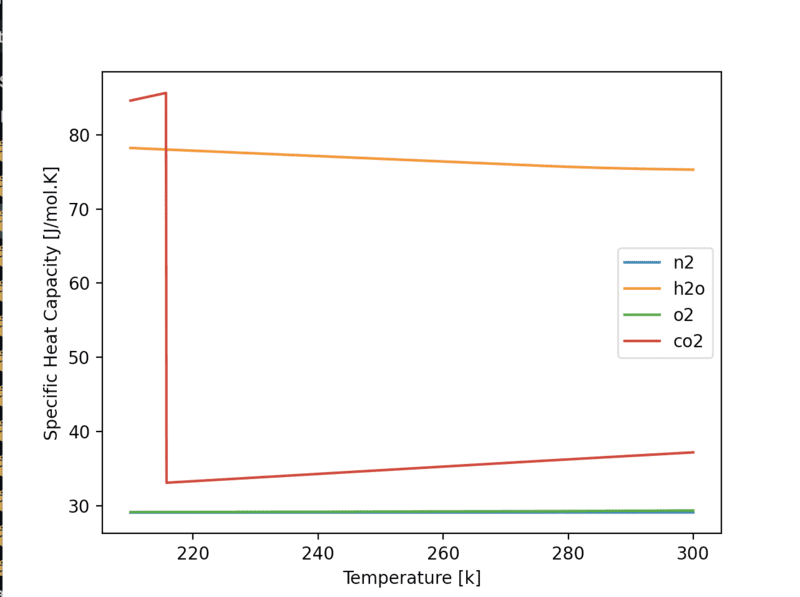The N2 and O2 heat capacities are almost identical (I don't think we have discussed the inclusion of O2 in this model however I've included it here). The CO2 heat capacity is also very similar to N2/O2 while in the gas phase. H20 heat capacity is not similar to the others however if N2/O2/CO2 take up a high enough mass fraction of the total mixture then this constant molar heat capacity assumption seems reasonable

The CO2 heat capacity will jump up when it transitions to solid phase. This plot however is actually the correlation used for the liquid phase (as thermo does not recognise solid CO2 it uses the liquid correlation instead). We can manually add solid correlations where needed. It doesn't look like we will need one here though given the constant molar heat capacity assumption

Did you notice a typo error in the heat balance equation in the paper (it is in the axial heat dispersion term)?
Hmm not an obvious one. Possibly you're looking at the derivative itself? The way they have written them, its really two first derivatives multiplied together, rather than a second derivative which is what it should be

Tomorrow I will rewrite the equations in terms of molar flows and mole fractions instead of mass flows and mass fractions, and propose some improvements to the formulation, particularly of the axial dispersion terms. I will also begin to formulate how to discretize the model so it looks much more like our tanks in series model.
Will converting to molar basis involve replacing each mass term with molar*mW term? The mW would then cancel out it seems and we would be left with the same equations except assuming a molar basis?

I am particularly interested to see this time how you discretise the model. Will we be going the route of assuming ##l## to be a multiple of ##\Delta x## in order to end up with favourable discretised equations?

Last edited: